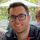cancel
Showing results for
Did you mean:Regular Visitor

## Multiply conditions return different results

Hi all,

New to dax here. I have one condition that I need to return values with multiplication and I was able to make it work with the syntax below

VAR Sample1 = CALCULATE ([Measure1], 'Account'[Account Level 1] = "A")

RETURN
[Measure1] * 0.5

Now I need to include another condition where if 'Account'[Account Level 1] = "B", return [Measure1]

How should I write it?

Thanks!

1 ACCEPTED SOLUTIONCommunity Support

Hi @fzhang ,

You could create a measure like below:

``````Measure =
IF (
SELECTEDVALUE ( 'Account'[Account Level 1] ) = "A",
[Measure1] * 0.5,
IF ( SELECTEDVALUE ( 'Account'[Account Level 1] ) = "B", [Measure1] )
)
``````

Best Regards,

Jay

Community Support Team _ Jay
If this post helps, then please consider Accept it as the solution
to help the other members find it.
2 REPLIES 2Community Support

Hi @fzhang ,

You could create a measure like below:

``````Measure =
IF (
SELECTEDVALUE ( 'Account'[Account Level 1] ) = "A",
[Measure1] * 0.5,
IF ( SELECTEDVALUE ( 'Account'[Account Level 1] ) = "B", [Measure1] )
)
``````

Best Regards,

Jay

Community Support Team _ Jay
If this post helps, then please consider Accept it as the solution
to help the other members find it.Super User

Hi @fzhang ,

I suggest you use SWITCH to define multiple conditions

For example:

SWITCH(
TRUE(),
'Account'[Account Level 1] = "A", [Measure 1] * .5,

'Account'[Account Level 1] = "B", [Measure 1]

)